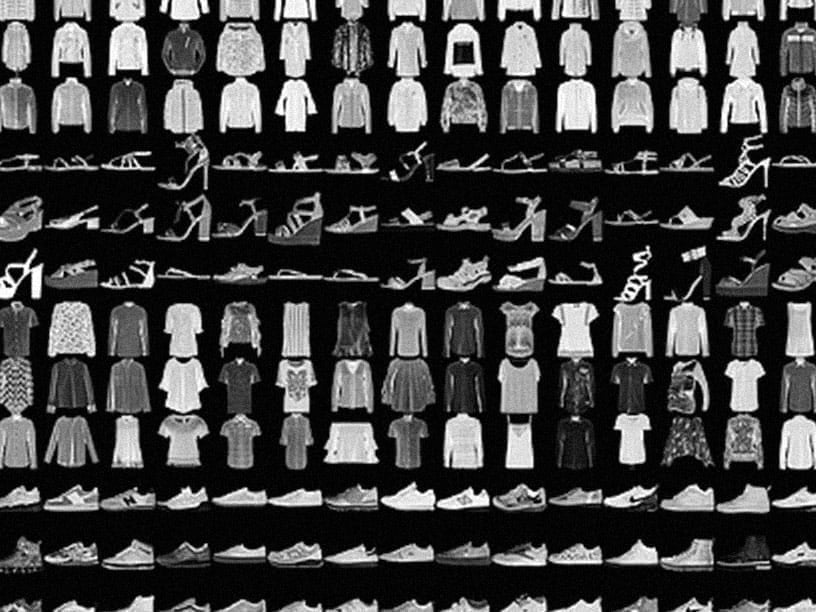# TensorFlow 是一个端到端开源机器学习平台#### 针对新手

```import tensorflow as tf
mnist = tf.keras.datasets.mnist

x_train, x_test = x_train / 255.0, x_test / 255.0

model = tf.keras.models.Sequential([
tf.keras.layers.Flatten(input_shape=(28, 28)),
tf.keras.layers.Dense(128, activation='relu'),
tf.keras.layers.Dropout(0.2),
tf.keras.layers.Dense(10, activation='softmax')
])

loss='sparse_categorical_crossentropy',
metrics=['accuracy'])

model.fit(x_train, y_train, epochs=5)
model.evaluate(x_test, y_test)
```

#### 针对专家

Subclassing API 提供了由运行定义的高级研究接口。为您的模型创建一个类，然后以命令方式编写前向传播。您可以轻松编写自定义层、激活函数和训练循环。请运行下面的“Hello World”示例，然后访问教程以了解详情。

```class MyModel(tf.keras.Model):
def __init__(self):
super(MyModel, self).__init__()
self.conv1 = Conv2D(32, 3, activation='relu')
self.flatten = Flatten()
self.d1 = Dense(128, activation='relu')
self.d2 = Dense(10, activation='softmax')

def call(self, x):
x = self.conv1(x)
x = self.flatten(x)
x = self.d1(x)
return self.d2(x)
model = MyModel()

logits = model(images)
loss_value = loss(logits, labels)
```

## 常见问题的解决方案## 新闻和通告2021 年 8 月 10 日2021 年 7 月 7 日
TensorFlow Hub 对现实世界的影响2021 年 5 月 19 日2021 年 5 月 19 日

[]
[]